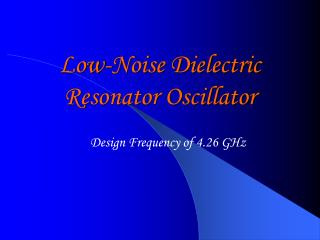DownloadDownload PresentationLow-Noise Dielectric Resonator Oscillator

# Low-Noise Dielectric Resonator Oscillator

Download Presentation## Low-Noise Dielectric Resonator Oscillator

- - - - - - - - - - - - - - - - - - - - - - - - - - - E N D - - - - - - - - - - - - - - - - - - - - - - - - - - -
##### Presentation Transcript

1. Low-Noise Dielectric Resonator Oscillator Design Frequency of 4.26 GHz

2. Introduction • Group Background • Radio Frequency Circuit Design • Microwave Frequency Circuit Design • Origin of Project • Suggested by Prof. Feng of High Speed Integrated Circuits Group

3. Objective • Dielectric Resonator Oscillator (DRO) • High frequency • Low phase noise • Purpose of DRO • Digital clock source • Current Issues • Jitter at very high frequencies

4. Objective • Advantages of DRO • Frequency stability • Tunable • High Quality factor (20,000 at 10 GHz) • Dielectric resonator minimizes noise • Low construction cost

5. Design

6. Characterization of 50 Ohm Microstrip Line • Copper Board Parameters: • Substrate : Ultralam • Line Parameters (after calibration): • L = 7.62 cm • W = 0.43 cm

7. Characterization of Dielectric Resonator • Utilization of Aluminum Casing for Measurement of Resonator • Measured Resonant Frequency : • f = 4.26 GHz • De-embed Measured Data

8. DC Bias Stub • Calculation of Bias Stub Lengths

9. DC Bias Stub • We want Zin to appear as an open circuit,

10. DC Bias Stub • Utilize ADS for Stub Length Optimization • DC Stub Capacitors = • DC Blocking Capacitors =

11. DC Bias Circuit • Active Bias Circuit establishes operating point for the transistor • PNP Transistor (Q1): 2N3906 Vbe = -0.75V , b1 = 100 • RF NPN Transistor (Q2): MRF571 Vce = 10V , b2 = 80

12. DC Bias Circuit Design • Specification • Vcc = 15V, Vce(Q2) =7V , Ic(Q2) =60mA

13. Characterization of Transistor • Obtain S-parameters using Network Analyzer

14. Negative Resistance Criteria for Oscillation • One-port negative resistance model

15. Optimization of Zin • Simulation Results

16. Optimization of Zin • Network Analyzer • Check at the Design Frequency, f=4.26 GHz

17. Optimization of Zin • Measured Results

18. Single Stub Matching Network • Smith Chart • accounts for Negative Resistance for Oscillation

19. Single Stub Matching Network Design • b= 1.92 • B = 1.92 / 50 Ohms = 0.0384 • jB = (1/Zos)(tan ((2pLstub)/ leff)) • Zos = 46.17 Ohms ( from ADS) • Lstub = 0.1682 l • l = 4.95 cm • Lstub = 8.3 mm • d= 0.4822 – 0.3142 = 0.168 l = 8.3 mm

20. Functionality Tests • Spectrum Analyzer • Checking for Oscillation and Phase Noise

21. Challenges and Solutions • Challenge: • DC blocking Capacitors on Microstrip Line • At very high frequencies, Capacitors look inductive • Solution: • Tried to couple the line * Tried 39pF Capacitors

22. Challenges and Solutions • Challenge: • DC Bias Circuit / Transistor with Gain • Problems with finding a suitable transistor with gain • Solution: • Rebuilt DC Bias Circuit to give more current * Chose Transistor with larger , MRF571.

23. Challenges and Solutions

24. Challenges and Solutions • Challenge: • Emitter Stub Length • Had trouble figuring out how to find emitter stub length for negative resistance • Solution: • Tried Trial and Error Method by starting with 2.6mm and cutting 2mm at a time to try to find optimal length and checked for stability in ADS. * Figured out how to use ADS variable optimization

25. Challenges and Solutions • Challenge: • Transistor Burnout • One of the two transistors apparently burned out while operating • Smelled something burning and checked current which ended up being 2.4 mA vs. 56 mA • Solution : • First replaced DC Bias circuit transistor and found that it was okay * Second replaced the RF Transistor.

26. Verification Methods • ADS Simulations • Between each step, ADS was used to simulate the circuit to find out if it would work ideally for that particular section of the circuit • In many cases, the simulations aided in finding problems that could have been potentially disastrous if we had built the board first

27. Recommendations • Use ADS to simulate your plan of action. • Measure each components parameters. • Make sure the Transistor is bias at the correct operating point to supply enough gain for oscillation to occur. • Take Design Frequency into account at all times ( i.e., capacitors are high frequencies behave as inductors).

28. Fine